# How do I input operation symbols?

### Input rules for operations. Operations priority sequence.

The app supports the standard order of operations: parenthetical expressions, exponents (powers), multiplication and division, addition and subtraction. When the priority of two expressions is the same, the calculation is performed from left to right.

For addition, use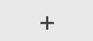.

##### Subtraction
For subtraction, use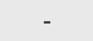.
Note: This key can also be used to input the minus sign before a negative value.

##### Multiplication
For multiplication, use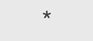.
In some cases a multiplication symbol can be omitted.

5*x can also be entered as 5x
3*(x - 5) can be entered as 3(x - 5)
x*(5 - x) can be entered as x(5 - x)
(x + 3)*(x - 5) can be entered as (x + 3)(x - 5)
2*x(4 - 8*x)*(6*x - 10)*5 can be entered as 2x(4 - 8x)(6x - 10)5

##### Division
For division, use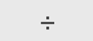.

The calculator supports division by numbers. The calculator does not support division by a variable.

Note: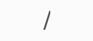symbol can be used for fractions input only. Do not use it for division.

##### Exponents
Exponents are typed using the power symbol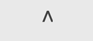.

Note: Exponents cannot be decimals or variables.

Exponents of variables or expressions with variables must be positive integers only:
x2 would be typed as x^2, (-x)4 would be typed as (-x)^4.
Expressions with variables: (5x)^2, (2x+4)^3, ((x-8)/3)^2.

Exponents of numbers and number expressions could be positive numbers, negative numbers or zero:
22 would be typed as 2^2
(-5)8 would be typed as (-5)^8
(
1/2
)
-3 would be typed as 1/2^-3 or 1/2^(-3)
(2.5 + 3.1)3 would be typed as (2.5 + 3.1)^3.

##### Parentheses
The calculator supports parentheses (), including nested parentheses.

Monday, December 4, 2023

Contact